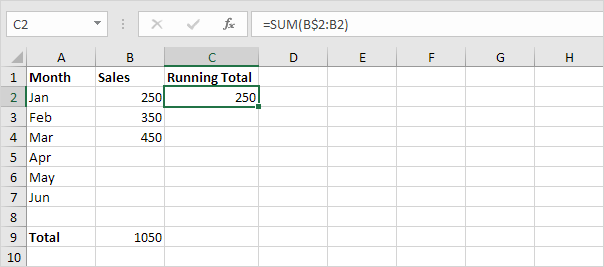# Running Total

This example teaches you how to create a running total (cumulative sum) in Excel. A running total changes each time new data is added to a list.

1. Select cell B9 and enter a simple SUM function.2. Select cell C2 and enter the SUM function shown below.Explanation: the first cell (B\$2) in the range reference is a mixed reference. We fixed the reference to row 2 by adding a \$ symbol in front of the row number. The second cell (B2) in the range reference is a normal relative reference.

3. Select cell C2, click on the lower right corner of cell C2 and drag it down to cell C7.Explanation: when we drag the formula down, the mixed reference (B\$2) stays the same, while the relative reference (B2) changes to B3, B4, B5, etc.

4. For example, take a look at the formula in cell C3.5. For example, take a look at the formula in cell C4.6. At step 2, enter the IF function shown below (and drag it down to cell C7) to only display a cumulative sum if data has been entered.Explanation: if cell B2 is not empty ( means not equal to), the IF function in cell C2 displays a cumulative sum, else it displays an empty string.

7. Enter the sales in April.Previous articleCapital Investment in Excel
Next articleActiveX Controls in Excel VBA